Fitting the Humphreys Distribution to a Dataset

by P Barber ( January 2010)

INTRODUCTION

1. A significant challenge with simulation modelling is to be able to determine the distribution of any resultant data set.

2. Packages such as Crystal Ball (http://www.oracle.com/appserver/business-intelligence/crystalball/index.html) or EasyFit (www.matwave.com) provide a means of determining the best-fit, from many of the common distributions available, but unfortunately the Humphreys distribution (see section 32) is not included within the libraries of these packages.

3. This paper sets out an iterative process for determining the NMX parameters and then goes on to outline a method of estimating these parameters directly from measurements of the dataset Mean, Standard Deviation, Skew and Kurtosis. ( see section 16)

ITERATIVE METHOD OF FITTING A DISTRIBUTION

4. The Iterative Method utilises the Anderson Darling test .

5. At the beginning of the iterative process an estimate of the NMX parameters of the data-set is made. In this example parameters of  N=126.479, M=170.162, X=198.597 have been assumed, and this results in an Anderson Darling statistic of AD = 108.08

 N = 126.479 M = 170.162 X = 198.597 AD = 108.083

6.  The value of the first parameter (N) is then increased by (0.001). The results of the first and second trial are shown below.

 N = 126.479 126.48 M = 170.162 170.162 X = 198.597 198.597 AD = 108.082605 108.114 Mvt AD= 0.031253

7.  From the above it can be seen that the effect has been to increase the value of AD by 0.031253, since it is desired to decrease the value of AD it is deduced that the value of the first parameter (N) should have been reduced, rather than increased.

 N = 126.479 126.478 M = 170.162 170.162 X = 198.597 198.597 AD = 108.082605 108.051 Mvt AD= -0.031247

8.  The process of reducing the value of (N) is then repeated until three iterations, providing four data elements, has been completed.

 126.479 126.478 126.477 126.476 170.162 170.162 170.162 170.162 198.597 198.597 198.597 198.597 108.082605 108.051 108.02 107.989 -0.031247 -0.031241 -0.031235

9.  The next stage is to plot the values of AD against the moving parameter (N), fitting a ‘Polynomial Order 2’ trendline and using the fitted equation to calculate the turning point.

The data we have is:

 N = 126.479 126.478 126.477 126.476 AD = 108.083 108.051 108.02 107.989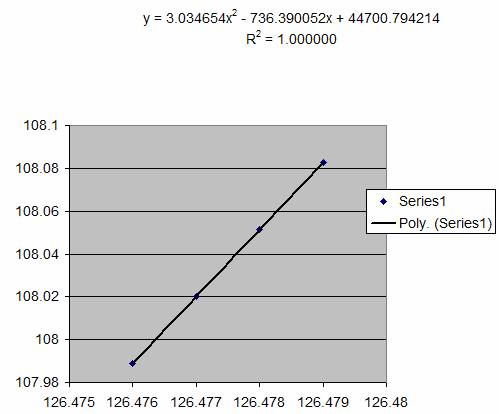10.  The equation of the trendline is of the form:

Y = aX2 + bX + c

And the turning point occurs when dY/dX = 0

Differentiating the equation above we have:

dY/dX = 2aX +b = 0

Hence the turning point occurs when:

X = -b / 2a

The values found in the fitted trendline were:

a = 3.034654

b = - 736.390052

and if we substitute these into the equation:

X = -b / 2a

We get X = 736.390052/(2 x 3.34654) = 121.330

11.  Setting N = 121.330 we get a results which reduces the AD statistic from 107.988 to 17.5667

 N = 126.479 126.478 126.477 126.476 121.33 M = 170.162 170.162 170.162 170.162 170.162 X = 198.597 198.597 198.597 198.597 198.597 AD = 108.082605 108.051 108.02 107.989 17.5667 Mvt AD= -0.031247 -0.031241 -0.031235 -90.4222

12.  Holding the parameters N and X constant the process is then repeated with M.

 N = 121.33 121.33 121.33 121.33 M = 170.162 170.161 170.16 170.159 X = 198.597 198.597 198.597 198.597 AD = 17.566721 17.5463 17.526 17.5056 Mvt AD= -0.020384 -0.020368 -0.020352

13.  With the four data elements being

 M = 170.162 170.161 170.16 170.159 AD = 17.5667 17.5463 17.526 17.5056

Which when plotted: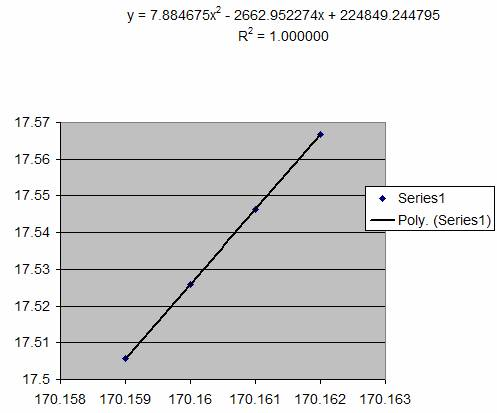and if we substitute the equation parameters into the turning point equation:

X = -b / 2a

We get X = 2662.952274/(2 x 7.884675) = 168.869

Insertion of this value results in a reduction in AD from 17.505617 to 4.4608879

 N = 121.33 121.33 121.33 121.33 121.33 M = 170.162 170.161 170.16 170.159 168.869 X = 198.597 198.597 198.597 198.597 198.597 AD = 17.566721 17.5463 17.526 17.5056 4.46089 Mvt AD= -0.020384 -0.020368 -0.020352 -13.0447

14.  The process is then repeated holding parameters N and M constant while adjusting X. Once four iterations have been completed the data is used to construct a trendline, calculate the turning point and reduce the value of AD further. Repeating the process until no further reduction can be achieved.

 X = 198.597 198.596 198.595 198.594 AD = 4.46089 4.45963 4.45838 4.45713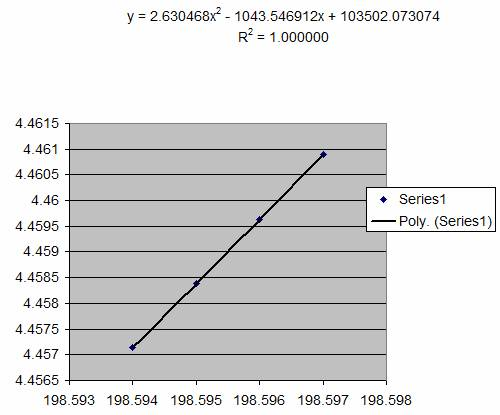and if we substitute the equation parameters into the turning point equation:

X = -b / 2a

We get X = 1043.546912/(2 x 2.630468) = 198.358

Which when substituted yields a further reduction in the value of AD.

 N = 121.33 121.33 121.33 121.33 121.33 M = 168.869 168.869 168.869 168.869 168.869 X = 198.597 198.596 198.595 198.594 198.358 AD = 4.460887915 4.45963 4.45838 4.45713 4.31148 Mvt AD= -0.001257 -0.001251 -0.001246 -0.145657

15.  While it can be seen that the above method provides a method of fitting the NMX parameters of the Humphreys Distribution to a data-set, the method involves a significant number of iterations to achieve a final convergence, particularly since the rate of convergence declines with each iteration, as can be seen above. An alternative method, utilising the measurable parameters of the data-set: Mean, Standard Deviation, Skew and Kurtosis.

ALTERNATIVE MEANS OF FITTING A DISTRIBUTION

16.  The parameters Mean, Standard Deviation, Skew and Kurtosis of a data-set can be measured using standard Excel Functions. It should be noted however that the values of  Skew and Kurtosis vary slightly with variations in the size of the data sample. Skew is a measure of the third standardised moment of the data-set while Kurtosis is a measure of the fourth standardised moment. As the size of the data-set is increased more values are included ‘in the tails’ of the distribution, and these affect the calculation of these two variables. To overcome this problem a standard data-set with N= 10,000 elements was employed.

17.  Investigation of fitted data sets reveals that a number of relationships exist, namely:

a) Pm and Skew

b) (Mean – M)/standard deviation and Skew

c) (X – N)/standard deviation and Kurtosis

d) (X – N)/standard deviation and Skew

Pm and Skew

18. The relationship between Pm and Skew was found to be:(Mean – M)/standard deviation and Skew

19. The relationship between (Mean – M)/standard deviation and Skew is shown below.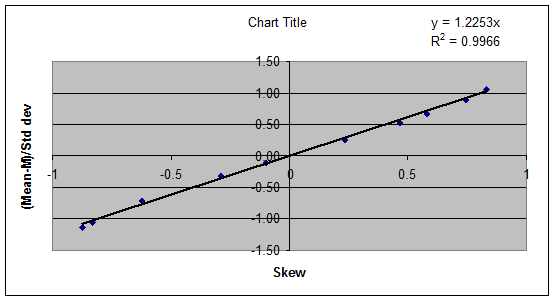(X – N)/standard deviation and Kurtosis

20. The relationship between (X – N)/standard deviation and Kurtosis is shown below.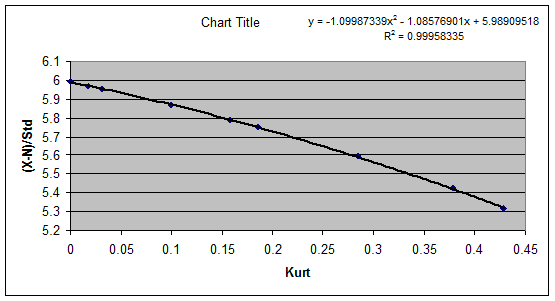(X – N)/standard deviation and Skew

21. The relationship between (X – N) / standard deviation and Kurtosis is shown below.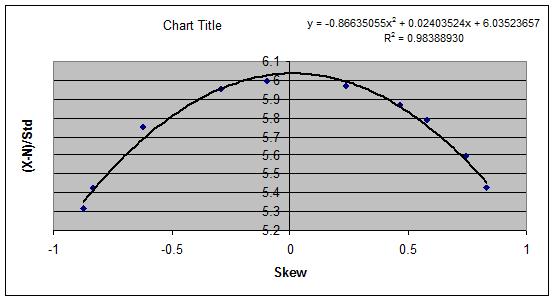22. Using the above equations it was possible to develop a set of lookup tables which would predict the NMX parameters for data-sets which possessed a Humphreys Distribution. Three lookup tables were established based upon Skew+1 (SKEW+1 to unsure that all the values in the table were positive) and these provided the following factors:

a) Pm

b) G = (Ave – M) / Std

c) H = (X – N) / Std

Where:

Ave = the Average or Mean of the data-set

Std = the Standard Deviation of the data-set

Estimates of the NMX Humphreys Distribution could then be estimated from the above equations as follows:

a) M = Ave – (G x Std)

b) N = M – (Pm x H x Std)

c) X = (H x Std) + N

23. Although the above relationships provide a means of predicting the NMX parameters for data-sets that possess a Humphreys Distribution, the above relationships were not sufficient to provide the best-fit, when the underlying distribution was different. A number of tests were carried out using data created with a Beta Distribution and the following additional relationships involving changes in Kurt were established.

24.  Firstly for data which can be fitted closely to a Humphreys Distribution, it was found that there was a relationship between Skew and Kurt, as shown below.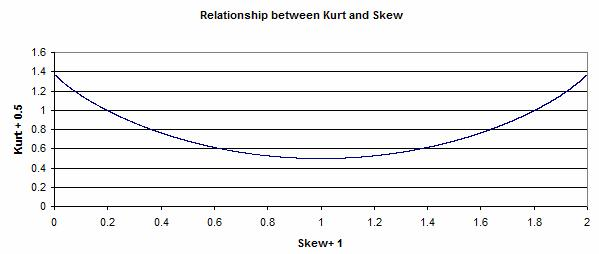25.  However, it was found that the relationship between Skew and Kurt changed when the data-set differed from the Humphreys Distribution. The plots below show how differences in the value of Kurt (dKurt) could be related to changes in the lookup table factors:

Pm,

G= (Ave – M) / Std and

H = (X – N) / Std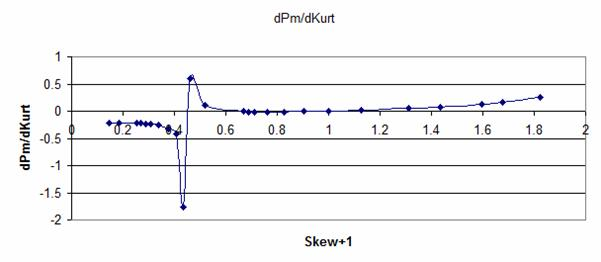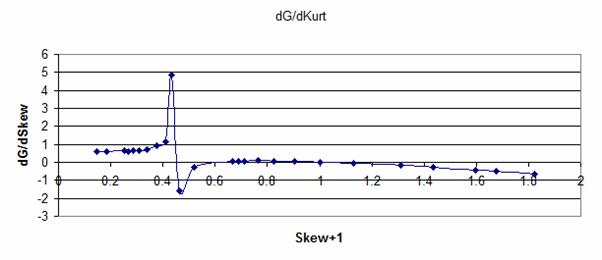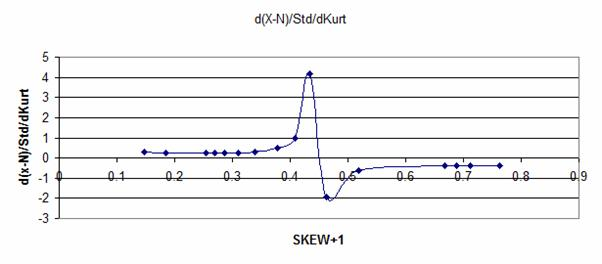26.  These relationships were used to create a lookup table which would predict the NMX parameters over a wider range of data-sets. However, it should be noted that in creating the lookup tables it was assumed that the relationships between data points was linear. With the limited number of data points employed in the trial it is therefore inevitable that some inaccuracy will occur. However, it is considered that the method provides a useful means of predicting the NMX parameters, which can then be checked using the Anderson Darling statistic and if necessary further iteration will yield a result close to the best fit.

APPLICATION OF LOOKUP TABLES

27.  The section below illustrates the process for estimating NMX parameters using the developed Lookup Tables. (down-load table)

The parameters for a data set have been measured as follows:

Mean (Ave)                =  123.0769

Standard Deviation    = 15.38512

Skew                          =  0.823478

Kutosis                       = 0.278257

Then (Skew+1) =   1 + 0.823478 = 1.823478

And (Kurt+0.5) =  0.5 + 0.278257 = 0.778257

From this value two lookup reference values are calculated, given by:

a) Look1 = Round((Skew+1) – 0.0005,3)  = 1.823

b) Look2 = Round((Skew+1) + 0.0005,3) = 1.824

A segment of the table is shown below:Skew+1 to (X-N)/Std"

"

"

"

"

"

"

"

"

"

"

"

"

"

"

"

"

"

1.822

1.0265

5.5092

0.3519

1.6212

0.9384

-0.6387

-0.6610

0.1798

0.2465

1.823

1.0279

5.5074

0.3545

1.6212

0.9401

-0.6397

-0.6610

0.1791

0.2470

1.824

1.0294

5.5056

0.3536

1.6212

0.9417

-0.6410

-0.6610

0.1784

0.2500

1.825

1.0309

5.5038

0.3497

1.6212

0.9433

-0.6425

-0.6610

0.1778

0.2500

Reading from the second column in the table it can be seen that for a value of  (Skew+1) = 1.823 we would expect (Kurt+0.5) to be equal to 1.0279.

However the value of (Skew+1) we have is 1.823478 and we must therefore adjust for the difference between the measured value and the value in the table.

If we read the value of (Kurt+0.5) at (Skew+1)=1.824, then we find an expected value of (Kurt+0.5) of  1.0294.

Interpolating between these two values we have an expected value of (Kurt+0.5) of:

Expected (Kurt+0.5) = 1.0279 + (1.0294 – 1.0279)(1.823478 – 1.823)/(1.824 - 1.823)

=  1.028617

Now the value of (Kurt+0.5) that has been measured is 0.778257

Hence the difference between the measured and expected values of (Kurt+0.5) is

(0.778257 – 1.028617) = -0.25036

This difference of (Kurt+0.5)= -0.25036 will be used to adjust the values of Pm, H and G obtained from the table.

28.  The basic value of H=(X-N)/Std can be read from the third column in the table.

for Look1 = 1.823 we have H = 5.5074 and

for Look2 = 1.824 we have H = 5.5056

Given that the actual value of (Skew+1) was 1.823478

We need to interpolate to determine the basic value of H=(X-N)/Std as follows:

Basic value of H = 5.5074 + (5.5056 – 5.5074)(1.823478 – 1.823)/(1.824 - 1.823)

Giving a basic value of H=(X-N)/Std = 5.5065396

This basic value of H now needs to be adjusted to take account of the difference between the expected and the measured value of Kurt+0.5, as determined above.

The factor for adjusting the value of H with respect to the difference in Kurt+0.5 is provided in the fourth column of the table, we have, for:

Look1 H/KurtAdj = 0.3545 and for

Once again it is necessary to interpolate to determine the correct factor:

H/KurtAdj = 0.3534 + (0.3536 – 0.3545)(1.823478 – 1.823)/(1.824 – 1.823)

Producing an H/KurtAdj factor = 0.3529698

The estimated value of H is therefore = 5.5065396 + (0.3529698 x -0.25036)

Giving an estimate for H = 5.41817

29.  The basic value for Pm is found in the ninth column, reading at:

Look1= 1.823   Pm = 0.1791

A correction factor is found in the eighth column (slope) and is equal to -.0.6610

The calculation for adjusting, to determine the basic value of Pm is:

Basic Pm = 0.1791 + (1.823478 – 1.823) x -0.6610 = 0.17878

This basic value of Pm must now be adjusted to take account of the difference in Kurt+0.5)

The adjustment factor Pm/KurtAdj is found in the tenth column of the table, selecting values at:

Look1 = 1.823 , we have Pm/KurtAdj = 0.2470 and

Look2 = 1.824   we have Pm/KurtAdj = 0.2500

Interpolating to find the correction factor, we have:

Pm/KurtAdj factor = 0.2470 + (0.2500 – 0.2470)(1.823478 – 1.823)/(1.824 – 1.823)

Applying this factor to correct the value of Pm we have:

Pm Corrected = Basic Pm + (Pm/KurtAdj factor x diff Kurt+0.5)

Giving Pm corrected = 0.17878 + (0.24843 x -0.25036) = 0.11658

29.  The final factor to be determined from the table is G=(Ave-M)/Std, the Look1 value of G is extracted from the sixth column, and the adjustment slope from the fifth column, while the G/KurtAdj is read from the seventh column. Reading at Look1 = 1.823, we have:

Look1 G = 0.9401

Slope = 1.6212

Correcting to determine the basic value of G we have:

Basic G=(Ave-M)/Std = 0.9401 + (1.823478 – 1.823) x 1.6212 = 0.94087

The KurtAdj for G has to be obtained by interpolating between the two adjustment values, as follows:

G/KurtAdj  factor = -0.6397 + (-0.6410 + 0.6397)(1.823478 – 1.823)/(1.824-1.823)

Hence the G/KurtAdj factor = -0.64032

Adjusting the corrected value of G we have:

Estimate of G = Basic G + (G/KurtAdj factor x diff Kurt+0.5)

Estimate of G = 0.94087 + (-0.64032 x -0.25036) = 1.10118

30.  From the measured parameters of the data-set Skew and Kurtosis we have now determined the following estimated factors:

Pm(est) = 0.11658

H = (X-M/Std = 5.41817

G = (Ave – M)/Std = 1.10118

While the following factors were measured:

Mean = Ave = 123.0769

Std = Standard Deviation =  15.38512

The NMX parameters of the Humphreys Distribution can now be estimated as follows:

M = Ave – (G x Std) = 123.0769 - (1.10118 x 15.38512) = 106.135

N = M - (Pm x H x Std) = 106.135 – (0.11658 x 5.41817 x 15.38512) = 96.417

X =  N + (H x Std) = 96.417 + (5.41817 x 15.38512) = 179.776

31.  The data was fitted using the iterative method and a comparison of the results from the two methods is shown below:

 Parameter Iterative Method Method N = 96.417 96.395 M = 106.135 106.136 X = 179.776 179.744

THE HUMPHREYS DISTRIBUTION

32. Humphreys (1979) described a method of constructing a cumulative distribution curve, based on the three parameters: Minimum, Most Likely, Maximum (NMX). Unlike the PERT Distribution, which is based on the incomplete Beta Function, the Humphreys Distribution is based upon a Normal Distribution which is pulled and stretched about the Most Likely (M) point.

33.  The cumulative probability corresponding to the Most Likely (M) point is given by:

Pm = (M – N) / (X – N)

34.  The illustration below shows Cumulative Humphreys Distribution curves for a range of Pm’s.35.  The example below illustrates the construction of the Humphreys Distribution with the parameters:

 N= 100 M= 130 X= 200

36.  The first stage in the process is to calculate Pm.

 Pm = (M - N) (X - N)

37.  Inserting the parameters this gives:

 Pm = (130 - 100) = 0.3 (200 - 100)

38.  The next stage is to establish a reference probability scale. The intervals of this scale will depend be governed by the total number of data values, but will be generated by the sequence:

1/2N, 1/N + 1/2N, 2/N + 1/2N, 3/N + 1/2N, …..  (N-1)/N + 1/2N

39.  If the number of data values N = 100 then the reference probability sequence would be:

 0.005 0.015 0.025 0.035 " " " " 0.995

40.  In order to calculate the data points it is necessary to remap the reference probability sequence. The chart below illustrates the process.41.  The reference probability scale is shown on the horizontal axis, while the re-mapped scale is shown on the vertical axis. The Humphreys Distribution assumes that the distribution either side of the Most Likely point (M) is in the form of a normal distribution. The first part of the curve is covered by the range from zero to Pm. To construct the curve it is necessary to re-map this range from zero to 0.5 (the probability that at which the Mode occurs on a Normal Distribution). Re-mapping on this section of the curve is achieved by the formula:

For P < Pm           Pr = 0.5P/Pm

Where:

Pr = the re-mapped probabilities

P = the values of the reference probability sequence

Pm = the cumulative probability corresponding to the Most Likely point (M), calculated as shown above.

42.  The second part of the curve is a little more difficult, but the process can be followed by reference to the illustration above. The re-mapped probabilities in the second part of the curve begin at a value of  Pr = 0.5, so this is the first term in the equation. In order to re-map the values of P, which lie above Pm, it is necessary to deduct the value of Pm and then to adjust the proportions by multiplying by 0.5 and dividing by (1 – Pm). The equation for the upper part of the curve is shown below:

For P > Pm          Pr = 0.5   +  0.5 (P – Pm)/(1 – Pm)

43.  A sequence of data is shown below. Although the value of P = Pm = 0.5 does not appear in the reference probability sequence, it can be seen that, such a value would correspond to a Pr value of  Pr = 0.5.

 P Pr 0.005 0.0083 0.015 0.0250 0.025 0.0417 0.035 0.0583 " " " " " " 0.285 0.4750 0.295 0.4917 0.305 0.5036 0.315 0.5107 0.325 0.5179 " " " " 0.965 0.9750 0.975 0.9821 0.985 0.9893 0.995 0.9964

44.  The next stage in the process is to calculate the Normal Standard Deviates corresponding to the values of Pr. In Excel this can be achieved using the function:  =NORMSINV(B37)  Where B37 refers to the column and rows containing the values of Pr. A segment of the table is shown below:

 P Pr SD 0.005 0.0083 -2.39398 0.015 0.0250 -1.95996 0.025 0.0417 -1.73166 0.035 0.0583 -1.56892 " " " " " " " " " 0.285 0.4750 -0.06271 0.295 0.4917 -0.02089 0.305 0.5036 0.008952 0.315 0.5107 0.02686 0.325 0.5179 0.044776 " " " " " " 0.965 0.9750 1.959963 0.975 0.9821 2.100164 0.985 0.9893 2.300346 0.995 0.9964 2.690114

45.  The Humphreys Distribution assumes that the distance (M – N) = -3SD and the distance (X – M) = +3SD. The conversion of the SD values into values of ‘X’ is undertaken in two parts.

For P<Pm            Y  =  M  +  (M – N) SD/3

And for P>Pm     Y  =  M  +  (X – M) SD/3

 P Pr SD Y 0.005 0.0083 -2.39398 106.060 0.015 0.0250 -1.95996 110.400 0.025 0.0417 -1.73166 112.683 0.035 0.0583 -1.56892 114.311 " " " " " " " " " 0.285 0.4750 -0.06271 129.373 0.295 0.4917 -0.02089 129.791 0.305 0.5036 0.008952 130.209 0.315 0.5107 0.02686 130.627 0.325 0.5179 0.044776 131.045 " " " " " " 0.965 0.9750 1.959963 175.732 0.975 0.9821 2.100164 179.004 0.985 0.9893 2.300346 183.675 0.995 0.9964 2.690114 192.769

46.  It can be seen that the values of Y in the above table do not extend to N = 100 and X = 200 this is because the reference probability sequence contains only one hundred values and hence the probabilities of 0.00135 and 0.99865 are not covered. However, if a greater number of data points had been covered by the reference probability sequence, say 10,000, then it is likely that values below N = 100 and X = 200 would have been created.

47.  The Cumulative Frequency Distribution for the Humphreys Distribution, with N = 100, M = 130 and X = 200 obtained by plotting the values of P and Y from the above data, is shown below: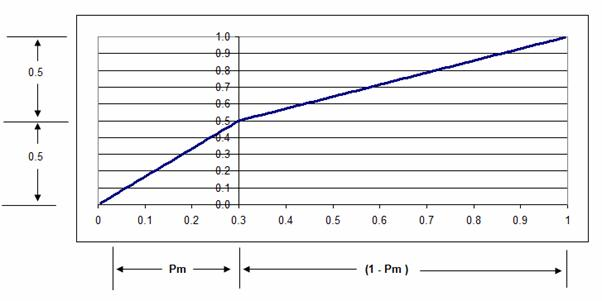48.  A bell curve, useful only for illustration purposes, can be produced for this data by utilising the Excel Function:

B  =NORMDIST(M33,0,1,FALSE)

Where:

B = the ordinates of the Bell Curve

M33 = Column and rows containing the values of SD

0 = zero = the value of the mean

1 = standard deviation = 1

False = to obtain the bell curve rather than a cumulative frequency curve

The Bell Curve is then obtained by plotting values of ‘Y’ against ‘B’

 Y B 106.0602 0.02272 110.4004 0.058445 112.6834 0.089076 " " 129.7911 0.398855 130.2089 0.398926 130.6267 0.398798 131.0448 0.398543 " " 179.0038 0.043968 183.6747 0.028305 192.7693 0.010702ALTERNATIVE TO USING EXCEL FUNCTIONS

49. The example above demonstrated the use of the Excel function =NORMSINV(Pr) to calculate a value of SD from Pr. It should also be noted that Excel provides other useful functions such as = GAMMAINV(Po, Alpha, Beta ) which will produce a cumulative Gamma distribution, = BETAINV(Po, Alpha, Beta, Lower bound, Upper bound) which will produce a Beta distribution, and LOGINV(Po, Mean, Standard deviation) which will produce a Lognormal distribution, should these be required. The results of these Excel functions are produced by an iterative process rather than by direct calculation.

50. Although the Excel library functions can be used in an Access database, it may be found more convenient, and certainly faster, to employ the equation set out below, which is shown in the “Handbook of Mathematical Functions With Formulas, Graphs, and Mathematical Tables” Edited by Milton Abramowitz and Irene A. Stegun published by the National Bureau of Standards, United States Department of Commerce, Applied Mathematics Series 55 June 1964. This equation only covers values of  P up to 0.5 so a little manipulation is necessary.

(P in the equation below is equal to Pr above)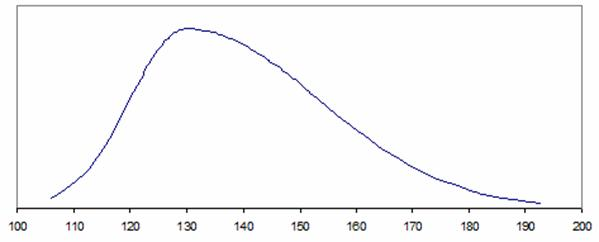THE  ANDERSON DARLING TEST

51. The Anderson Darling Test is an empirical test which is used in commercial applications such as Crystal Ball and is useful where it is necessary to test data against a number of alternative distributions.

52. The graphs below illustrate the discrimination that this test provides when fitting an NMX parameters of the Humphreys Distribution.53.  The graph above shows how the AD statistic increases significantly if a small movement is made in the value of one of the parameters, NMX, of the Humphreys distribution.

21. The figures in the graph above are based on the fitting of a distribution where N = 18,800, M = 20,000 and X = 22,800. hence a discrimination of  +/- 5 is equivalent to an accuracy of 2.5 x 10-4 .

54. The Anderson Darling Statistic is calculated as follows:

a) The data set comprising of ‘N’ data points (created using an Excel Beta Function with parameters Alpha=9, Beta=5, Min=100, Max=200) is ranked in ascending order as shown below. (the Rank column is used later so that data can be accessed as a lookup table)

 Rank Yi 1 117.2852 2 119.7510 3 121.0388 4 121.9360 " " " " 4998 164.9755 4999 164.9788 5000 164.9820 5001 164.9853 5002 164.9885 " " " " 9997 194.7800 9998 195.1447 9999 195.6451 10000 196.5454

b) An estimate of the Humphreys Distribution parameters is then made, in this case N=126.479, M=170.162, X=198.597 (which gives a Pm of (170.162 – 126.479)/(198.597 – 126.479) = 0,60572) This is not a particularly good fit, as shown in the illustration below, but it provides a starting point. The data is shown in blue and the Humphreys Distribution estimate is shown in red.c) The next stage is to calculate the Standard Deviates from the values in the data set, based on the assumed distribution. The following equations are used for this operation:

For Yi < M

SD = 3(Yi – M)/(M – N)

And for Yi > M

SD = 3(Yi – M)/(X – M)

The results are as shown below:

 Std Dev Rank Yi based on Yi 1 117.2852 -3.6314 2 119.7510 -3.4621 3 121.0388 -3.3736 4 121.9360 -3.3120 " " " " " " 4998 164.9755 -0.3562 4999 164.9788 -0.3560 5000 164.9820 -0.3557 5001 164.9853 -0.3555 5002 164.9885 -0.3553 " " " " " " 9997 194.7800 2.5973 9998 195.1447 2.6358 9999 195.6451 2.6886 10000 196.5454 2.7835

b) The next stage is to calculate the Cumulative Probabilities associated with each of these Standard Deviation values. Because the two parts of the Humphreys Distribution are based on Normal Distributions with different parameters, It is necessary to divide the calculations into two parts. The formula are as follows:

For Yi < M       Pi = NORMSDIST(SD) x Pm / 0.5

For Yi > M       Pi =  Pm + (NORMSDIST(SD) – 0.5) x (1 – Pm) / 0.5

Values are produced as follows:

 Std Dev Pi for Rank Yi based on Yi NMX Fit 1 117.2852 -3.6314 0.000171 2 119.7510 -3.4621 0.000325 3 121.0388 -3.3736 0.000449 4 121.9360 -3.3120 0.000561 " " " " " " " " 4998 164.9755 -0.3562 0.437145 4999 164.9788 -0.3560 0.437246 5000 164.9820 -0.3557 0.437347 5001 164.9853 -0.3555 0.437448 5002 164.9885 -0.3553 0.437550 " " " " " " " " 9997 194.7800 2.5973 0.996295 9998 195.1447 2.6358 0.996690 9999 195.6451 2.6886 0.997171 10000 196.5454 2.7835 0.997880

55.  Note that the logic behind the Anderson Darling Test is not entirely clear (personal comment – meaning that the author does not understand why it works), but appears to be related to the fact that if the cumulative probabilities of the data and the fitted distribution are plotted against each other, then if the assumed distributions fits the data exactly, then the resultant plot will be a straight line. The relevant plot is shown below: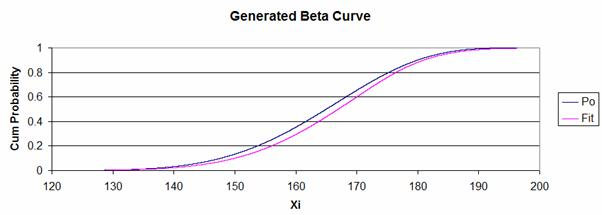56.  The plot of Pi against PO is shown in red, while a straight line has been added in black to demonstrate how the linear requirement is not met by the parameters selected in the example. Note that the values of PO have been calculated by the Rank sequence and are in the form:

1/2N, 1/N + 1/2N, 2/N + 1/2N, 3/N + 1/2N, …..  (N-1)/N + 1/2N

Where N = the number of data elements (this process is described in section 38)

c) The next stages in the process are to calculate the natural logarithms of Pi and (1 – Pi) these are shown below:

 Std Dev Pi for Rank Yi based on Yi NMX Fit Ln(Pi) Ln(1-Pi) 1 117.2852 -3.6314 0.000171 -8.675105 -0.000171 2 119.7510 -3.4621 0.000325 -8.032430 -0.000325 3 121.0388 -3.3736 0.000449 -7.707516 -0.000450 4 121.9360 -3.3120 0.000561 -7.485499 -0.000561 " " " " " " " " " " " " 4998 164.9755 -0.3562 0.437145 -0.827491 -0.574732 4999 164.9788 -0.3560 0.437246 -0.827259 -0.574913 5000 164.9820 -0.3557 0.437347 -0.827028 -0.575092 5001 164.9853 -0.3555 0.437448 -0.826797 -0.575272 5002 164.9885 -0.3553 0.437550 -0.826565 -0.575453 " " " " " " " " " " " " 9997 194.7800 2.5973 0.996295 -0.003712 -5.598116 9998 195.1447 2.6358 0.996690 -0.003315 -5.710816 9999 195.6451 2.6886 0.997171 -0.002833 -5.867693 10000 196.5454 2.7835 0.997880 -0.002122 -6.156329

d) The values of  Ln(1 –Pi) are then reversed, such the associated with the first element of data (the one which has the lowest value in the set) there is the value of Ln(Pi) and set along-side, the value taken from the last data element for Ln(1 – Pi) as shown below.

 Pi for Reverse in reverse Rank Yi NMX Fit Ln(Pi) Ln(1-Pi) Rank Order 1 117.2852 0.000171 -8.675105 -0.000171 10000 -6.156329 2 119.7510 0.000325 -8.032430 -0.000325 9999 -5.867693 3 121.0388 0.000449 -7.707516 -0.000450 9998 -5.710816 4 121.9360 0.000561 -7.485499 -0.000561 9997 -5.598116 " " " " " " " " " " " " " " 4998 164.9755 0.437145 -0.827491 -0.574732 5003 -0.575632 4999 164.9788 0.437246 -0.827259 -0.574913 5002 -0.575453 5000 164.9820 0.437347 -0.827028 -0.575092 5001 -0.575272 5001 164.9853 0.437448 -0.826797 -0.575272 5000 -0.575092 5002 164.9885 0.437550 -0.826565 -0.575453 4999 -0.574913 " " " " " " " " " " " " " " 9997 194.7800 0.996295 -0.003712 -5.598116 4 -0.000561 9998 195.1447 0.996690 -0.003315 -5.710816 3 -0.000450 9999 195.6451 0.997171 -0.002833 -5.867693 2 -0.000325 10000 196.5454 0.997880 -0.002122 -6.156329 1 -0.000171

In the last column it can be seen that the value of -6.156329 which appears as the Ln(1-Pi) for item 10000 is now associated with the first item.

e) An elemental statistic is then calculated, for each row, by adding the values Ln(Pi) and ‘Ln(1 – Pi) in reverse Order’ and multiplying this line sub-total by (1 – 2i)/N (where i = Rank)

 Ln(1-Pi) in reverse Rank Ln(Pi) Order AD Stati 1 -8.675105 -6.156329 0.001483 2 -8.032430 -5.867693 0.004170 3 -7.707516 -5.710816 0.006709 4 -7.485499 -5.598116 0.009159 " " " " " " " " 4998 -0.827491 -0.575632 1.402422 4999 -0.827259 -0.575453 1.402291 5000 -0.827028 -0.575272 1.402161 5001 -0.826797 -0.575092 1.402029 5002 -0.826565 -0.574913 1.401898 " " " " " " " " 9997 -0.003712 -0.000561 0.008543 9998 -0.003315 -0.000450 0.007528 9999 -0.002833 -0.000325 0.006315 10000 -0.002122 -0.000171 0.004586

The calculation for the fourth element is as follows:

AD Stat(N=4) = (Ln(Pi) – ‘Ln(1-Pi)in reverse’)(1 – (2Rank)/N

AD Stat(N=4)  =   (-7.485499 -5.598116) ( 1 – (2 x 4))/10000

AD Stat(N=4) =  ( - 13.083616 )( - 7)/10000 = 0.009159

f) The elemental statistics are then summed, and ‘N’ deducted from the total, to produce the Anderson Darling Statistic (AD).

 Ln(1-Pi) in reverse Rank Ln(Pi) Order AD Stati 1 -8.675105 -6.156329 0.001483 2 -8.032430 -5.867693 0.004170 3 -7.707516 -5.710816 0.006709 4 -7.485499 -5.598116 0.009159 " " " " " " " " 4998 -0.827491 -0.575632 1.402422 4999 -0.827259 -0.575453 1.402291 5000 -0.827028 -0.575272 1.402161 5001 -0.826797 -0.575092 1.402029 5002 -0.826565 -0.574913 1.401898 " " " " " " " " 9997 -0.003712 -0.000561 0.008543 9998 -0.003315 -0.000450 0.007528 9999 -0.002833 -0.000325 0.006315 10000 -0.002122 -0.000171 0.004586 Sum 10,108.08

AD = Sum of elements – N = 10,108.08 – 10,000 = 108.08

An AD of 108.08 indicates that the distribution selected, of N=126.479, M=170.162, X=198.597 does not fit the data..

57. It should be noted that with a normal distribution the points P = 0 and P = 1 correspond to + and – infinity. In creating a data it has been assumed that values of Pi will be selected from the equivalent mid class points, as such the values of Pi selected correspond to the series: 0.5/n, 0.5/n + 1/n, 0.5/n + 2/n, …..etc. where n equals the number of data points to be selected. For n = 10,000 the series would be of the form 0.00005, 0.00015, 0.00025, etc upto 0.99995

58. It should also be noted that it is assumed value of N and X occur at -3 and +3 standard deviations, corresponding to probabilities of 0.00135 and 0.99865. From the above it will be apparent that a number of the values in the data set will lie outside the N and X limits.

REFERENCES

Humphreys G. (1979) Turning Uncertainty to Advantage, McGraw-Hill Book Company (UK) Limited. P22-30